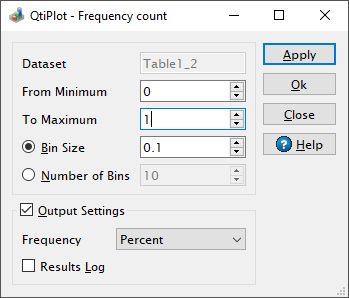# Frequency Count Dialog

This dialog is activated by selecting the Frequency Count command from the Analysis -> Descriptive Statistics menu. It can be used in order to calculate the frequency distribution of the data values from a table column.

Figure 5-83. The Frequency Count dialog.Internally QtiPlot sorts the input data and detects the minimum and the maximum values which are displayed in the From Minimum and To Maximum input boxes. Then it creates a set of bins in this range using the bin size specified in the Step Size box. When pressing the Apply button QtiPlot counts the number of times a data value falls within a bin. Data values equal to the low-edge of a bin are included in the bin while values equal to the upper-edge of the bin are excluded from it, but they are counted in the next bin (if any).

The results are placed in a newly created table which contains four columns with a row for each bin. The values displayed in these rows are: the center value of the bin, the upper limit of the bin, the frequency count for the bin and the cumulative frequency up to that bin, respectively.